Search IntMath
Close

450+ Math Lessons written by Math Professors and Teachers

5 Million+ Students Helped Each Year

1200+ Articles Written by Math Educators and Enthusiasts

Simplifying and Teaching Math for Over 23 Years

# IntMath Newsletter - Complex numbers, Pi Day, Formula sheets

By Murray Bourne, 07 Mar 2009

7 March, 2009

1. Math Tips - Complex Numbers
2. Pi Day - 3/14
3. Latest IntMath Poll - Math formula sheets
4. From the Math Blog
5. Final Thought - Math is human, after all

## 1. Math Tips - Complex Numbers

Try this on your calculator: √−5.

You calculator showed an error, right? Hopefully this reminded you of what your math teacher always said — you can’t find the square root of a negative number. This is because whenever you multiply any number by itself, you get a positive number.

For example, 3 × 3 = 9, and −3 × −3 = 9

Since squaring a number always gives a positive number, we normally conclude that it’s not possible to find the square root of a negative number.

But is this correct?

During the 16th and 17th centuries, mathematicians were interested in solving polynomial equations (because of their importance in science and engineering), something like:

x3 + x2x + 2 = 0

But there was a problem. There should be 3 solutions for the above equation, but they could only find 1 solution.

Why do we expect 3 solutions? Let's look at 3 cases where we know something about the roots.

Tip: A "root" of an equation is a value that makes the equation "true".

(a) A linear equation has one solution (eg x + 2 = 7 has solution x = 5). The highest power of x in a linear equation is 1.

In this image, we see the graph of a linear equation and it cuts the x-axis in one place only (marked with a red dot).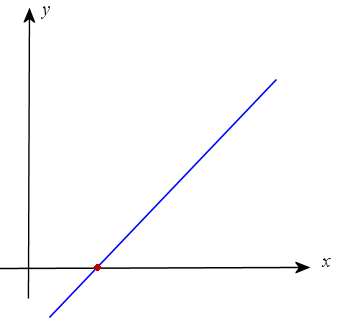Tip: Wherever the graph cuts the x-axis will give you the root of an equation.

[Exceptions: If the linear equation has slope 0, it will be parallel to the x-axis and will not pass through it, so will have no solutions, like the following image.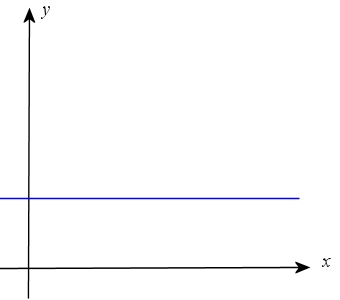The other exception is if the line is parallel to the y-axis.]

Tip: Always draw the graph! The Chinese are right - a picture is worth a 1000 words. When you draw graphs you get a much better idea of what is going on.

(b) A quadratic equation has 2 solutions (eg x2 − 4x + 3 = 0 can be factored giving solutions x = 1 or x = 3.) The highest power of x in a quadratic equation is 2.

Here we see a typical quadratic equation with 2 roots (marked with red dots).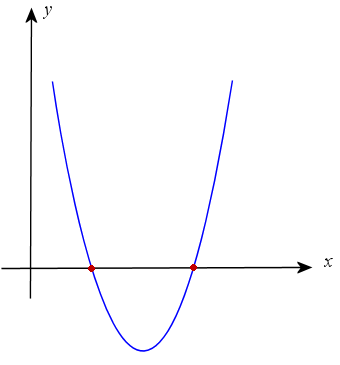We can also have the case where there is only one solution. This might occur if our quadratic is of the form (x − 3)2 = 0.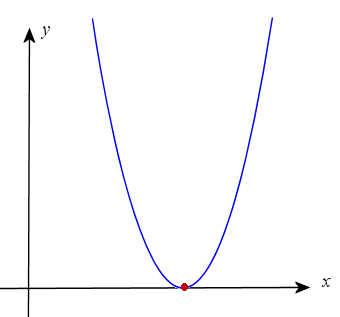But sometimes when you use the quadratic formula you will get a negative number under the square root.

For example, when we solve x2 + x + 1 = 0 using the quadratic formula, we get:

x = [−1 ±√(1 − 4)] / 2

= [−1 ±√(−3)] / 2

At this point we usually give up and say there are "no real solutions". But what does this really mean? We obtained 2 solutions, as expected, but they are "illegal".

The graph of this situation is as follows. The curve does not cut through the x-axis at all so there appear to be no solutions.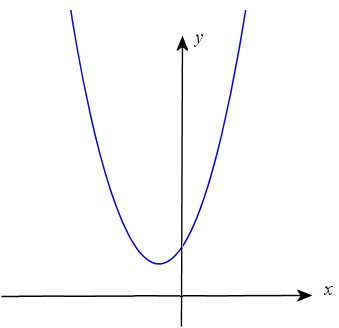(c) We expect a cubic equation to have 3 solutions since the highest power of x is 3. In the following image, we see that the curve cuts the x-axis in 3 places, giving us 3 roots.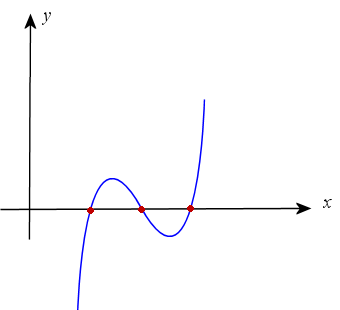But sometimes, there is a repeated root, so we only have 2 places where the curve cuts the x-axis.

An example here could be (x − 1)(x − 3)2 = 0.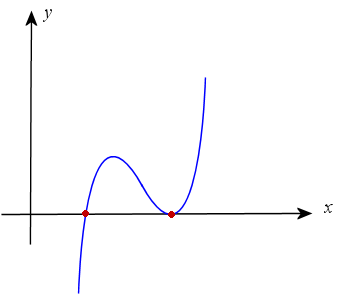Back to our first cubic equation example, x3 + x2x + 2 = 0. The mathematicians could find one solution OK (x = −2), but where were the other 2?

The graph of this situation is as follows. There is one point where the curve cuts through the x-axis.By the early 1600s, the famous mathematician and philosopher Rene Descartes called the square root of a negative number "imaginary". No-one was really sure if they were legitimate numbers, but because such numbers kept cropping up in different fields of mathematics — and they were found to "work" — they were studied more closely.

Around this time, the letter i (possibly for "imaginary") was used to denote the square root of −1. So we write:

i = √−1

A complex number contains a real part and an imaginary part.

Using this, we can solve our cubic equation from before. We cannot see the other 2 solutions but we can apply various methods to find the complex roots and we get:

x = (1 ± i√3) / 2

So there are 3 roots after all, x = 2 and x = (1 ± i√3) / 2.

In 1734 the brilliant Swiss mathematician Leonhard Euler produced the following formula:

(cos θ + i sin θ)n = cos nθ + i sin nθ

This formula was enormously powerful for calculating values of complex numbers and it integrated trigonometry and complex numbers.

In the years since the 18th century, complex numbers have been regarded as legitimate numbers in the field of mathematics. They have proved to be important in electronics.

You can see more about these special numbers, with applications in electronics, here: Complex Numbers.

As an interesting diversion, complex numbers are also involved in art. Fractals are made using complex numbers. Here's an example:Image source.

See more on this topic: Fractals.

## 2. Pi Day

Coming up on March 14th is Pi Day, so named because the first 3 digits of pi and the date 3/14 coincide. Some people go all out and have a special event at 1:59 PM, to recognize the next 3 digits of pi.

Pi is an interesting number and mathematicians have spent countless hours over the centuries learning more about it.

• Pi is irrational, which means it has an infinite number of decimal places. Here is the value of pi showing the first 20 decimal places: 3.1415926535 8979323846... Go here to see the first million digits of pi.
• The Greek mathematician Euclid in the 3rd century BCE showed that pi is always the circumference of a circle divided by its diameter, no matter the size of the circle. He used a method that was a forerunner of calculus.
• You can get an approximation for pi by dropping needles on a piece of paper. How? Watch out for an article on this coming up in the squareCircleZ blog on Pi Day.

What is your school doing on Pi Day? If you don't have anything planned yet, there are some good ideas in these resources:

## 3. Latest IntMath Poll - Math formula sheets

A poll during Feb 2009 asked readers if they were allowed to use formula sheets during their tests and exams. I asked this question because in a previous poll many students said that one of the main reasons that math is difficult because learning math formulas is hard.

Poll results:

36% Some exams only
32% Never
31% Always

Tip: Even if you know you're going to get a formula sheet in your exam, learn your formulas well enough so that you don't need the sheet.

Why?

Because if you know the formulas well, you are more likely to choose the correct one. I have seen many students rely 100% on the formula sheet and it is obvious that they have no idea which formula to use for a particular situation.

Of course, you can always check that you have the formula exactly right using the sheet you are given.

The latest poll asks readers what they do on the night before a math exam. Do you study until late, or go to bed early? You can answer on any page in Interactive Mathematics.

## 4. From the Math Blog

1) Friday math movie - math problem solving with mind maps
This week's movie gives you some good tips on how to solve math problems.

2) Akamai - visualizing Web traffic data

3) Friday Math Movie - Math in Art
This movie shows some beautiful art that comes from the study of dynamical systems in math.

4) Manga Guides to Statistics and Databases
Here's a series of math books based on Japanese manga (comics).

5) Energy reduction with Google's help
How much electricity is your home consuming right now? Not sure? Here’s a neat tool from Google that will allow you to see details of your daily energy consumption.

## 5. Final Thought - Math is human, after all

Here's a great quote:

“Concern for man and his fate must always form the chief interest of all technical endeavors. Never forget this in the midst of your diagrams and equations.”
Albert Einstein

I realized some time ago that many math classes have no human element to them. That is, they are all algebra with no application and no implications for people.

So my suggestion for you, whether you are a student or a teacher, is to always look for what's behind the math that you are doing.

Math wasn’t invented to torture you — it was developed to solve some human problem. Go find what that problem was — you'll understand the math better and find it more interesting, too. (Most chapters in Interactive Mathematics start with some applications and/or history of the topic.)

Until next time, enjoy whatever you learn.

### 7 Comments on “IntMath Newsletter - Complex numbers, Pi Day, Formula sheets”

1. Marg-tata says:

WELL, THANK YOU VERY MUCH FOR THE INFORMATION YOU HAD GIVEN ME. IT WILL REALLY HELP ME MORE ABOUT KNOWING MATH.

2. Mohamed Kani says:

Hi sir,
It is very usefull for me. Thanks for sending this

3. Hurley says:

Your inmath newsletter is so helpful in my quest to finally develop my algebra and calculus skills.

Would appreciate receiving any financial-business related developmental problems. Thank you.

4. EBUNOLUWA says:

Thank you very much sir. I'm grateful. God bless you.

5. Max says:

Hello Murray,

Just went through the news letter , EXCELLENT , keep them coming , thanks,

All the best Max .

6. nandan says:

fantastics

7. Yaseer says:

This is amazing! Keep it up.

### Comment Preview

HTML: You can use simple tags like <b>, <a href="...">, etc.

To enter math, you can can either:

1. Use simple calculator-like input in the following format (surround your math in backticks, or qq on tablet or phone):
a^2 = sqrt(b^2 + c^2)
(See more on ASCIIMath syntax); or
2. Use simple LaTeX in the following format. Surround your math with $$ and $$.
$$\int g dx = \sqrt{\frac{a}{b}}$$
(This is standard simple LaTeX.)

NOTE: You can mix both types of math entry in your comment.